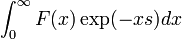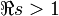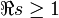# Wiener-Ikehara theorem

In mathematics, the Wiener-Ikehara theorem relates the behaviour of a real sequence to the analytic properties of the associated Dirichlet series. It is used in the study of arithmetic functions and yields a proof of the Prime number theorem. It is an example of a Tauberian theorem.

It was proved by Norbert Wiener and his student Shikao Ikehara in 1932.

## Statement

Let F(x) be a non-negative, monotonic decreasing function of the positive real variable x. Suppose that the Laplace transform$\int_0^\infty F(x)\exp(-xs)dx \,$

converges for$\Re s >1$ to the function f(s) and that f(s) is analytic for$\Re s \ge 1$, except for a simple pole at s = 1 with residue 1. Then the limit as x goes to infinity of exF(x) is equal to 1.

##  Application

An important number-theoretic application of the theorem is to Dirichlet series of the form$\sum_{n=1}^\infty a(n) n^{-s}$ where a(n) is non-negative. If the series converges to an analytic function in$\Re s \ge b$ with a simple pole of residue c at s = b, then$\sum_{n\le X}a(n) \sim c \cdot X^b$.

Applying this to the logarithmic derivative of the Riemann zeta function, where the coefficints in the Dirichlet series are values of the von Mangoldt function, it is possible to deduce the prime number theorem from the fact that the zeta function has no zeroes on the line$\Re (s)=1$.